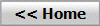Custom Search

COMPASS For Windows
 • Compass Tour • Other New Features • Cartography Tools • Live 3D Cave Images • On-line Help (New) • Written Description • Revision History: 99-18 • Testimonial Letters • Contact Author
Getting COMPASS
 • Getting Compass • Compass On A CD.
 • Installation Instructions • Antivirus Issues • Authenticating Files • False Positives
Registration Information
 • Credit Card Reg. • Reg. Info/Forms • Printable Reg. Form • 3rd Party Reg. Form
Auxiliary Tools/Information
 • Cave Data • 3D Glasses • ESRI/ArcView Tools
Tutorials - (New)
 • Inkscape Tips and Tricks • Radio Locations • Magnetic Anomalies • Installing Under Win8 • Compass on A Mac • Google Earth Overlay • Declination/Convergence • Cartography Tools • Sketch Editor. • SVG Export • Inkscape SVG Maps • Illustrator SVG Maps • SVG Round Tripping • Adopting SVG Maps • Complex Plot Tutorials • Trouble Shoot CaveX • Exporting HPGL • Canvas Digital Map • ArcView/ESRI • Italian Tutorial • Using the Compass CD • DEM Tutorial
 • Cave Related Links • User's Pages • User's Group • User Questions
Documents/White Papers
 • Compass File Formats • ArcView/ESRI • Finding Blunders • Finding Loops • Loop Closure • Least Squares Papers • Least Squares Issues • Passage Modeling • Survey Blunders • J. Halleck- On Loops • History/Philosophy • SEF File Format (1992) • About The Author
COMPASS For DOS
 • DOS Compass Demos • DOS Compass Features • Magazine Review
MISC.
 • Other Products

 BLUNDER LOCATION  Larry Fish  In 1979 I started working on a project to computerize the survey data for Groaning Cave. The cave had been discovered in 1969 and I was suddenly handed ten years of survey data that had never been processed by a computer. The early surveyors had processed all the information by hand and as a result, there many anomalies and problems in the data.  When I looked at the Groaning data, I found that many loops near the front of the cave had large loop closure errors. Luckily, I had more than one survey for some of the worst loops and was able to compare good surveys to bad surveys of the same passage. Much to my surprise, the errors in the surveys were not due to the accumulation of small, random errors; but were due to major blunders like reading the wrong end of the compass needle, transposing digits on the survey notes, mixing up azimuth and elevation, etc. By comparing good surveys to bad, I could easily fix a bad survey by repairing the worst blunders one by one until the survey closed.  As I played with the idea more, I discovered that I didn't always need a good survey to find the blunders in a bad survey. I could see that each blunder leaves its own special mark on the closure error. For example, an azimuth error has no effect on the vertical component of the closure error, so a compass error can never produce a vertical error. If you have a survey with one blunder in it, the blunder will produce a very specific kind of closure error. For example, let say you have a 10 foot length blunder on a shot that has an azimuth of 130 degrees and inclination of -10 degrees, the closure error will be 10 feet on an azimuth of 130 degrees and an inclination of of -10 degrees. To find the blunder, all you have to do is find a shot that matches that measurement.  You can of course try to find matches by hand, but this is very tedious. In addition, matching azimuth and inclination is much more complicated. It is much more useful to locate the errors using a computer. As a result, the COMPASS cave survey software has special routines that can locate blunders in survey loops.  UNDERSTANDING SURVEY ERRORS  In order to understand the process of locating blunders, it is useful to understand something about survey errors. There are three kinds of errors that occur in a cave survey:       1. Random Errors.       2. Systematic Errors.       3. Gross Errors or Blunders. 1. Random Errors. Random errors are generally small errors that occur during the process of surveying. They result from the fact that it is impossible to get absolutely perfect measurements each time you read a compass, inclinometer or tape measure. For example, your hand may shake as you read the compass, the air temperature may affect the length of the tape, and you may not aim the inclinometer precisely at the target. There are literally hundreds of small variations that can affect your measurements. In addition, the instruments themselves have limitations as to how accurately they can be read. For example, most compasses don't have line markings smaller than .5 degrees. This means that the actual angle may be 123.4 degrees, but it gets written down as 123.5.  All these effects add up to a small random variation in measurement of survey shots. Even though these errors are random, they tend to follow a pattern. The pattern is called a ”normal“ distribution and it has the familiar ”bell“ shaped curve. As a result of this pattern, we can predict how much error there should be in a survey loop if the errors are the result of small random differences in the measurements. If a survey exceeds the predicted level of error, then the survey must have another, more profound kind of error.   2. Systematic Errors. Systematic errors occur when something causes a constant and consistent error throughout the survey. Some examples of systematic error are: the tape has stretched and is 2 cm too long, the compass has five degree clockwise bias, or the surveyor read percent grade instead of degrees from the inclinometer. The key to systematic errors is that they are constant and consistent. If you understand what has caused the systematic error, you can remove it from each shot with simple math. For example, if the compass has a five degree clockwise bias, you simply subtract five degrees from each azimuth.  3. Blunders. Blunders are fundamental errors in surveying process. Blunders are usually caused by human errors. Blunders are mistakes in the processing of taking, reading, transcribing or recording survey data. Some typical blunders are: reading the wrong end of the compass needle, transposing digits written in the survey book, or tying a survey into the wrong station.   Blunders are the most difficult errors to deal with because they are inconsistent. For example, if you read the wrong end of the compass needle, the reading will be off by 180 degrees. If you transpose the ones and tens digits on tape measure, the reading could be off by anything from 0 to 90 feet.   THE ACCUMULATION OF SURVEY ERRORS   As you survey around a loop, the errors slowly accumulate, which effects how well the loop closes. It is important to understand how survey errors accumulate, because it allows us to make predictions about how well each loop should close. If you know how a loop should close, you can assess the quality of the survey measurements and the accuracy of the map as a whole.  Let's look at the way errors accumulate as you survey a cave. Let's say that you have a series ten foot shots and you have a ruler that is only accurate to plus or minus one foot. That means that sometimes you will read nine feet, sometimes ten feet and sometimes eleven feet and sometimes in between. As a simple example, here are the results of three length measurements:    Result:             A   B  C    Measurement:        9  10  11    Error:             -1   0  +1 Notice that the error is minus one, zero and plus one. If you combine the errors from two shots something very interesting happens. First since there are three results for each shots, there are a total of nine combinations:      A + A'    =    -1 + -1     =    -2      A + B'    =    -1 +  0     =    -1      A + C'    =    -1 + +1     =     0        B + A'    =     0 + -1     =    -1      B + B'    =     0 +  0     =     0      B + C'    =     0 + +1     =     1      C + A'    =     1 + -1     =     0      C + B'    =     1 +  0     =     1      C + C'    =     1 + +1     =     2 As we add together all the possible errors from two shots, the error begins to spread out. The lowest combined error is -2 and the highest combined error is +2. If we count how many shots fall into each error total, we get the following chart:                       -2  -1   0                           -1   0  +1                                0  +1  +2                       ------------------- Total In Each Range:  1    2   3   2   1   Notice how the results tend to concentrate in the middle and thin out toward the edge. If you combine enough shots in this way, then we will get the familiar ”bell shaped“ curve. This also gives us the probability of various total errors at the end of a three shots.        1  chance  in 9  the error will be -2.      2  chances in 9  the error will be -1.      3  chances in 9  the error will be  0.      2  chances in 9  the error will be +1.      1  chances in 9  the error will be +2.   This kind of information is very useful for determining the quality of a loop. For example if you get a loop error of minus three for the example survey, the chances are less than one in nine that it is a random error. Thus, it is very useful to be able to predict the kind of errors you should find in a loop.   TWO DIMENSIONS   So far, we have only looked at errors in one dimension. If we added in the error caused by the compass reading, the error becomes two dimensional. For example, lets say that you have a ten foot shot and 45 degree compass bearing. Lets also say that length is accurate to plus or minus one foot and the compass is accurate to plus or minus ten degrees. This will produce an error pattern that looks something like a rounded trapezoid: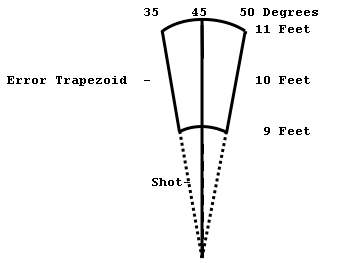If we combine the errors trapezoids for two shots the way we did in the first example, the two trapezoids will add and overlap. As before, most of the combinations will cluster in the middle and thin out toward the edge. This forms a kind of three dimensional bell shaped curve.   Another thing that happens with errors from two measurements is the values vary depending on the angle of the shot. For example, lets say that we have a shot with a compass angle of zero. If there is any uncertainty in the angle, then we will get errors in the east/west direction. Likewise, if the compass angle is 90, then errors will be in the north/south direction. Finally, if the shot is at 45 degrees, the errors will be equally north/south and east/west. As a result, we have to take into account the angle of each and every shot, before we can make a prediction about the loop error.  THREE DIMENSIONS   The same principles that we have used for two measurements apply to three. Using compass, length and inclination makes the potential errors cluster in a sort of spherical shape, and most of the results will concentrate around the center.   LOCATING BAD LOOPS.   Since we know the kind of pattern that random errors produce, we should be able to detect those situations where the errors more than random. In other words, we should be able to single out those loops that have errors that are likely caused by blunders. To do this, we need to make a prediction about the size of error we expect to see. If the actual error is greater than this amount, then the loop probably has a blunder in it.   To make a prediction about the size of error we expect, we need to know how accurately each instrument can be read. For example, if you can read a compass accurately to within one degree, every azimuth reading will have an ”uncertainty“ (or ”variance“) of about one degree. Each type and brand of compass, tape measure and inclinometer has a different level of ”uncertainty“ associated with it. For example, experiments on a test survey courses show that Suuntos are more accurate than Sustecos and Sustecos are more accurate than Silvas. Also, some surveyors are better than others and some caves are more difficult than others. All this adds up to varying levels of uncertainty in the survey measurements.   If you combine all these uncertainties around the loop, you will get a prediction of what the total error should be if all the errors are random. Notice, that each shot has a different effect on the outcome. For example, if the shot is heading north, a one degree azimuth uncertainty adds to the east/west errors. If the shot is heading east, the uncertainty adds to north/south errors. If you have loop with more shots in the north/south direction, you would expect the loop to have more east/west error. For this reason, every measurement in every shot should be used to make a prediction of the error. The resulting prediction is the ”standard deviation“ for the loop. Since the errors in a loop should be random (unless there is a blunder or systematic error), standard deviation is a good way to predict whether the loop has a blunder. The best way to understand standard deviation is to look at a graph: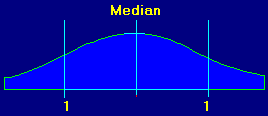This is a graph of a typical set of random events. Again, this is the famous ”bell shaped“ curve. It gives a visual representation of how the events are distributed. The events could be things like rain fall in Florida, wins on a roulette wheel or cave survey measurements. The center of graph is called the median and it is the average of all the events. You will notice that like our survey errors most of the events fall into the middle of the graph. Also, as you move to either edge, there are fewer events. At the bottom the graph, standard deviations are marked. They are numbered going away from the center. This is because we care about how far a particular measurement is from the average.   Standard deviations are a measure of how much variability there is in the data. The more variability, the larger the standard deviation will be. If you look at the second graph above, Here is another graph: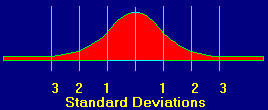You will notice that the data is more tightly clustered around the center. This means that the data has less variability. You can see that the standard deviations are smaller. If this were cave data, the tight clustering would mean that the cave was surveyed more carefully or with better instruments.   When we are looking for loops with blunders, we look for loops whose errors fall on the edge of the bell shaped curve. For example if we have a loop whose error falls ”three standard deviations from the mean,“ we would be very suspicious that the loop had a blunder. In other words, the standard deviation for a loop gives you a range of values that can be expected if the errors are random. LARGE ERRORS AREN'T ALWAYS BLUNDERS   Another important aspect of random errors is that over the course of a loop, the errors tend to cancel each other out. For example, sometimes you will read the compass half a degree positive, sometimes half a degree negative and sometimes right on. If you add up all these positives and negatives, the resulting error will be relatively small.   There is however, a small chance that the errors will fall in such a way that there will be a larger error. Sometimes, by random chance, most your compass readings will end up half a degree negative. This will give you a larger error even though there is no real blunder. It's like flipping a coin. Most of the time, you expect 50% head and 50% tails, but every once in a while, you will get five straight tails in a row. If you get five straight compass readings that are half a degree low, the error will be disproportional large.   So another way of understanding errors is to look at them as odds and chances. As the standard deviation goes up, the odds of the loop being blundered goes up. For example, if the standard deviation is 2, there is 5 chances in 100 that it is not a blunder. But if the error is 3 standard deviations, there are only 3 chances in 1000 that it is not a blunder.   The chart below gives more exact numbers for various standard deviations.   Standard Deviation    Percent Inside    Percent Outside         0.5                   38.30 %            61.70 %       1.0                   68.26 %            31.74 %       1.5                   86.64 %            13.36 %       2.0                   95.44 %             4.56 %       2.5                   98.76 %             1.24 %       3.0                   99.74 %             0.26 % You can see that if you have a loop with no blunders and only random errors, the loop error should fall within one standard deviation 68 percent of the time. By looking at the number of standard deviations a loop is away from our predicted error value, we get a clear idea about the quality of the loop. In simple terms, here is what the numbers mean:   BETWEEN 0 AND 1: If the value is between 0 and 1 the loop is of very high quality and probably does not have any blunders or systematic errors.   BETWEEN 1 AND 2: If the value is between 1 and 2, the loop is still probably good, but there is a greater chance the errors are caused by blunders.   GREATER THAN 2: If the value is greater than 2, there is a high probability that the loop has a systematic error or a blunder.   You can also make some inferences about the kind of blunder you have by looking at the size of the error:   Small Errors.  1 - 5 Standard Deviations.   Errors between one to five standard deviations generally are seen when there is a misreading of the instruments. For example, you could get this type of reading if you misinterpreted an azimuth of 320 as 330.   Medium Errors.  3 - 20 Standard Deviations.   Errors between three to twenty are seen with shot reversal. For example, if you read the wrong end of the compass needle or do a back sight without reversing the stations. This would give a reading that is incorrect by 180 degrees. The size of the errors is governed by the length of the shot. Long shots produce bigger  errors.     Large Errors.  15 - 100 Standard Deviations.   Errors greater than fifteen standard deviations are seen in situation where a survey has been tied into the incorrect the incorrect station. For example, if a survey is supposed to connect to station B12 and instead is connected B13, a large error will probably result. The size of the error will depend on how far the erroneous station is from the correct station.   These numbers are very general rules of thumb. Actual error can vary depending on the exact circumstances. For example, if you have a shot reversal on a very short shot, the error could easily be less than one standard deviation.   LOCATING INDIVIDUAL BLUNDERS.   The whole point of looking at the standard deviations of loops is to find loops that probably have blunders in them. After you have located loops with large deviations, the next step is to analyze the loop looking for the specific measurement that has caused the blunder.   The basic concept of blunder location is fairly simple: If you have a blunder and you fix it, the loop error should go down significantly. Thus, the trick to finding blunders is to find a measurement that when adjusted, makes the loop error go down significantly. The way to do this is to go around the loop adjusting each measurement, one at a time, trying to get the most improvement for that particular measurement. The adjustment that makes the biggest improvement in the loop error is the most likely candidate to be the blunder for that loop.  Since you adjust each measurement one at a time, the adjustment is constrained by the other measurements in the shot. As a result, there is a limit to how much improvement each adjustment can produce. For example, if you test-adjust the azimuth of a 10 foot shot, the result must fall on a circle with a 10 foot radius. This constrains the possible improvement to the circle. If the measurement is not the blunder, it very unlikely that the adjustment will significantly improve the loop error. This means that blunders leave unique signatures on the loop error.   By going through the survey and adjusting each measurement in turn, you will find that most adjustments make only modest improvements in the loop error. However, if you adjust the blundered measurement, it will produce a dramatic improvement in the loop error. In other words, the best adjustments will always correspond with the worst blunders.   BLUNDER SIGNATURES   Another way of looking at blunders is that they leave a signature on the error value. For example, lets say that you have made a blunder on a length measurement so that the shot is ten feet too long. This will add ten feet to the loop error. More important, the ten foot error will be in a direction that matches the azimuth and inclination of the shot. If the shot had an inclination of 10 degrees and an azimuth of 173, the error would be 10 feet in the direction of 173 azimuth, 10 degrees inclination. This creates a blunder signature that is unique. This means that only shots with same azimuth and inclination could cause the error that we see. As a result, it is often possible to zero in on the exact measurement that caused the blunder.   The signatures are often unique because there are two measurements that leave their mark on the signature. In our example, the combination of azimuth and inclination controls the signature. The odds of having these two measurements match exactly in a different shot are fairly low.   Even when you have an error signature that matches two or more shots, all is not lost. When two or more shots produce a signature that matches the error, you cannot be sure which shot has caused the error. However, you still have narrowed the number of likely candidates.   Again, the actual process of locating blunders is done by examining each and every measurement looking to see if it matches the error signature. You save the most successful adjustments. Since these are adjustments that do the best job of fixing the error, they are most likely candidates for blunders.   BLUNDER SENSITIVITY   There are several things that effect how well you can find blunders.   1. Loop Quality. Blunder location works best where the quality of the rest of the loop is high. If the unblundered shots in a loop have a high random error level, the blunders become overwhelmed by the accumulated random errors around the loop. The following table shows the sensitivity level vs. error level:      Unblundered    Length         Azimuth         Inclination    Error Level    Sensitivity    Sensitivity     Sensitivity     0 - .3 STD    1 Foot         1 Degree        1 Degree    .3 -  1 STD    5 Feet         5 Degrees       5 Degrees       >  1 STD    >10 Feet       >10 Degrees     >10 Degrees 2. Large Blunders vs Small Blunders. Large blunders are usually easier to detect than small ones. However, this can be tricky. For example, a 180 degree error may seem large, but if it is on .5 foot shot, the resulting error is less than a foot. 3. Multiple Blunders. If you have two or more blunders in a loop, each individual is more difficult to isolate. Two blunders on the same shot are almost impossible to isolate. If there are two blunders on different shots, one blunder tends to dominate and obscure the other.   4. Unique Signatures. The more unique the blunder is, the more likely it will be isolated. For example, lets say you have a blunder on a shot with a compass reading of 180 degrees. In addition, lets say that there are no other shots that come to within 45 degrees of the blunder. This blunder will be so unique that it can be isolated even in a relatively low quality loop. On the other hand, if you have many shots with a similar azimuth, random errors in the rest of the loop can cause the program to select several shot as the possible blunder shot. If you see several candidates with the similar ”Change“ values and similar improvement ratios, it may indicate that the blunder is not unique enough to be isolated. You can however, narrow it down to a few candidates. 5. Blunder location works slightly better on small loops. As the loop grows longer, random errors make a larger contribution to the error. Eventually, the accumulation of random errors becomes large enough so that the effect of the blunder is lost.  INTERSECTS   Once you have a list of good blunder candidates, there is another technique you can use to narrow your choices. The technique is called”Intersects.“ If a blundered shot participates in two loops, it should have a similar blunder signature in both loops. If it doesn't, you can pretty much eliminate it as a blunder candidate. Likewise, if the shot participates in more than two loops, it should have a similar blunder signature in all the loops. The only exception is the case where one of the other loops has an additional blunder which masks the original blunder. Even so, a blunder should show up with the same signature in most of the loops. This technique is very useful because it can eliminate candidates and narrow the field to just a few shots.   To give you an idea how intersects work, I have included some actual cave data from Lechuguilla cave showing two potentially blundered shots.    From   To    Measure    Change   New Error   New Dev. Improvement EPB1    GZ1    Length  21.49 Ft.      3.95       0.91         5.54 EPB1    GZ1    Length  20.36 Ft.      4.29       1.04         4.85 EPB1    GZ1    Length  24.34 Ft.      5.57       1.05         4.48 EPB1    GZ1    Length  24.40 Ft.      6.70       1.25         3.78 EPB1    GZ1    Length  23.18 Ft.      6.68       1.21         3.61 EPB1    GZ1    Length  21.85 Ft.      7.80       1.55         2.98 GZ4     GZ5   Azimuth -68.91 Deg      1.99       0.46        10.98 GZ4     GZ5   Azimuth -67.01 Deg      2.51       0.61         8.28 GZ4     GZ5   Azimuth -77.08 Deg      3.24       0.61         7.71 GZ4     GZ5   Azimuth -76.00 Deg      4.36       0.79         5.54 GZ4     GZ5   Azimuth -77.80 Deg      4.64       0.87         5.45 Notice that the first shot participated in different six loops, the second shot participated in five different loops. Also, notice that the ”change“ value is remarkably consistent between all the different loops. This means that the we are finding the same signature in completely different loops. This is highly indicative that the shot is a blunder.   BAD TIE-INS  One of the most common types of blunders occur when a shot is tied to the wrong station. This can happen through typos, misplaced stations in the cave and renaming stations after the survey is complete. Connecting a shot to the wrong station can cause very large survey errors.   It is possible to locate bad tie-ins, by breaking a loop at one of the stations in the loop. This allows the point at the break to move to its natural location. If there is bad tie-in, this point will move back toward the proper station. If the loop was incorrectly tied at the location of the break, the distance between the broken shot and the correct tie-in will be near zero. Thus the process for locating a bad tie-ins is to break the loop at every station around the loop and then keep track the closest connections.  With a list of the best candidates for bad tie-ins, the obvious typographical errors are easy to see. For example, here are some obvious examples of mis-ties in the Lechuguilla data set that were located using the technique:         OLD TIE    NEW TIE    OLD ERROR    NEW ERROR               K6       K6!    174.64 ft.      6.19 ft.          ECKJ11   ECKJ'11    152.78 ft.      1.75 ft.            BNM4      DNM4    127.52 ft.      3.44 ft.           EY52a     EY52A     87.47 ft.      5.39 ft.  As you can see, all of these mis-ties are due to obvious topographical or clerical errors.  DIFFICULT CASES   The blunder indications are not always as clear cut as the example described above. In many instances, there will be several good candidates for blunders. This can make it difficult to isolate the specific measurement that has caused the blunder. In these situations, it helps to have more insight into the finding process. Here are some of the most important issues: 1. Looks for loops that have errors greater than one standard deviation.   2. Look for ”Changes“ that cause high ratios of improvement.   3. Look for situations where there are multiple changes to the same measurement with similar values. For example, if you see four shots with 10 foot changes to the length, chances are very good that there is a 10 foot error in one of those shots.   4. Look for situations where most of the changes are to the same type of measurement. For example, if all the changes are to the azimuth measurement, then it is very likely that there is a blunder in the azimuth.   5. Look for situations where two or more loops share the same shot. If both loops indicate a blunder on the same shot and measurement, chances are very good that a blunder exists in that shot.   THE BLUNDER ALGORITHM   The actual technique for locating blunder involves complicated three dimensional geometry. If you are not mathematically inclined, you may want to skip this section.   The actual blunder location process consists of two steps: 1. Locating blundered loops. 2. Locating blundered measurements.   1. Locating Blundered Loops.   As stated above, the process of locating blunders in survey loops involves making a predictions about the size of error you should find if a loop contains random errors and no blunders. The key is making the prediction. The process of deriving the prediction is fairly straight forward. Basically, the program starts with a standard deviation for compass, tape, and inclination. The user can enter any value he chooses for these values. The program then uses the standard deviation for each instrument on each shot to calculate standard deviations for each shot in the loop. All these deviations are combined to get a deviation for the whole loop. This is the prediction.   It is important to apply the standard deviations to each shot individually because each shot has a different effect on the overall standard deviation. For example, if you have a loop with lots of north-south shots, the standard deviation in the azimuth will produce more east-west deviation.   The process of applying standard deviations to each shot is fairly simple. With standard trig, you calculate the Cartesian standard deviations for each shot. You then calculate the sum of all the squares of the standard deviation. This is the variance for the loop, and the square root of the variance is the standard deviation for the loop. In order to validate these concepts, algorithms and routines, I wrote a simulation program. The program works by building a simulated cave survey about 100 shots long. The azimuth, length and inclination of each shot is randomly selected. The program then traverses the survey repeatedly, generating random Gaussian distributed errors for each measurement in each shot. Each random Gaussian distributed error is scaled to match the standard deviations assumptions for each measure. At the end of each traverse, the program calculates the resulting error. After accumulating 100 error sets, I calculate the standard deviation of the errors. The program also uses the techniques I described above to make predictions about the same loop. This way, I can compare the predictions against the actual standard deviations simulated loop. At least in this setting, the routines accurately predict the standard deviations I find in the simulated loops. 2. Locating Blundered Measurements.   Once you have located loops that exceed the predicted error levels, you can zero in on the actual measurement that has caused the blunder. The technique for zeroing in on individual blunders involves finding an adjustment that makes the best improvement for each measurement in a shot. The trick is to find simple routines that calculate the best adjustments for azimuth, inclination and length measurements. The following section describes the algorithms for finding the best adjustment for each measurement. AZIMUTH ADJUSTMENT   Figure 3 represents a closed cave survey loop. Point S is the start of the survey, point E is the end of the survey. The angle at A1 is the angle we are going to adjust. The object is to find how much to change angle A1 to produce the best closure.If we rotate angle A1 through 360 degrees, point A2 will describe a circle whose radius is equal to the distance between point A1 and point A2. Since the azimuth angle measurements in cave surveying are referenced to magnetic north, point A2 tends to be a hinge point. That is, as A1 is rotated, the angle at A2 also changes but every angle at every shot from A2 to the end of the survey stays the same. As a result, as A1 is rotated, E describes a secondary circle whose center is offset from A1.   The secondary circle is important because it represents all possible points that E can occupy as we adjust angle A1. Thus, the best closure must be somewhere on it's circumference.   In figure 4, C represents the center of the secondary circle. This center C is located by taking the offset between A2 and E and adding it to A1. To find the best closure, we must find the point on the circle that is closest to the starting point S. Since the shortest distance between any point and a circle must be on a radius, the best closure must be at the point where the line C - S intersects the circle.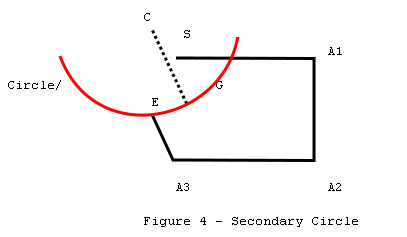We know the locations of S,E,A1,A2,C and the radius, so all of the remaining points and angles can be calculated from this information.   In figure 5, we construct a horizontal line from C to H; and using point S, we construct a right triangle. The location of G, the point of best closure, can be located using the triangle and polar coordinates. The new closure error is the distance between G and S, and the adjustment angle is the difference between angle G-C-H and angle E-C-H.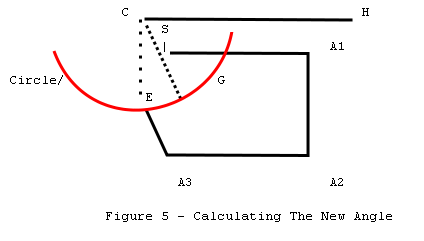LENGTH ADJUSTMENT Adjusting length is more complicated because the shot length has both the azimuth angle and inclination angle effecting it. As a result, the shot length is actually a vector in three dimensional space. Adjusting a particular shot for best closure is, in some ways, similar to adjusting azimuth. For example, figure 6 represents a plan view of the survey, where S represents the start of the survey and E the end of the survey. The survey has a large survey error. If we attempt to minimize the closure error by adjusting the shot length of A1-A2, point E will move, drawing line L which represents all possible adjustments of the shot. Obviously, the best adjustment will give the shortest distance between point S and line L.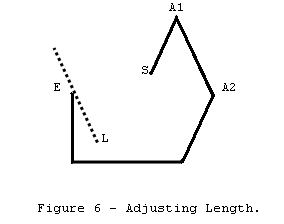Since we are working in three dimensions, it is easiest to work using vector algebra. The shortest distance between a point and a line in 3-D space is given by the following formula:  d=|U x V|   In other words, the distance from a line to a point is equal to the length of the cross product of the unit vector U and vector V. In this case, V is the vector E-L and U is the unit vector for line A1-A2. Since A1-A2 and E-L are parallel, we define E-L from A1A2:   L=DXi + DYj +DZk   From this we find the unit vector:   U =  EL / |EL|   The distance is then calculated using the cross products:    d= |U x ES|   Once we have found d, we have two sides of a right triangle and all other dimensions can be easily calculated.  INCLINATION ADJUSTMENT   Adjusting the inclination angle is the most difficult part of the closure process. Since it is an angular adjustment, it is similar to the azimuth adjustment except that it takes place in three dimensions. This is because the inclinometer is free to rotate around the vertical axis, where as the compass is always held in the horizontal plane.   Figure 7 is a profile plot of a survey. The vertical or Z axis is toward the top of the page. Point S represents the start of the survey and point E represents the end of the survey. As you can see, there is a closure error between S and E.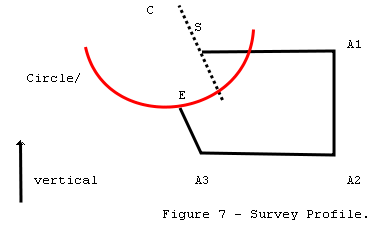In this example, A1 will be the inclination angle that will be adjusted. As with the azimuth adjustment, when A1 is rotated through 360 degrees, A2 describes a circle with A1 as the center. Since all of the shots from A2 to E remain unchanged, as the adjustment is made, A2 will hinge and E will move through a secondary circle offset from A1. Since the secondary circle represents the result of all possible adjustments, the best closure must be some point on the secondary circle. The task is to find the closest point on the circle to the starting point S. This will be the best possible closure we can attain by adjusting the dip angle at A1.   Since the secondary circle and the starting point S are in three dimensions, the process of finding the closest point is more complex.   Figure 8 represents a three dimensional view of the secondary circle. Point S is the start of the survey and point E is the end of the survey.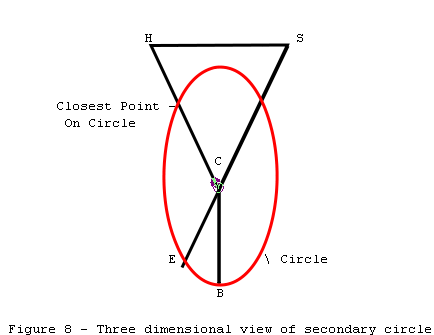The strategy for finding the closest point on the circle to S is to find the closest point from S to the plane of the circle. In the diagram, H is the closest point in plane of the circle to S. Now we calculate the intersect between H-C and the circle. This will be the closest point to S and will represent the best possible adjustment for this shot.   Since we know the location of E and can calculate the location of B and C, this gives us two vectors in the plane of the circle: C-B and C-E. By taking the cross product of CE and CB, we get a vector perpendicular to the plane. Taking the dot product of C-S and the unit vector of the perpendicular, we get the distance between S and the closest point on the plane: point H. Adding a perpendicular vector of length H-S to the coordinates for S gives us the coordinates for point H. With these coordinates, all other coordinates, angles and distances can be calculated.   In order to clarify the adjustment process, I have included the actual subroutines used in my blunder detection programs. The code is written in Turbo Pascal.   The code sample consists of three subroutines TESTAZM, TESTLEN and TESTINC which correspond with each measurement in a shot. For example, ”TESTAZM“ finds the best adjustment for the azimuth of a shot.   Each subroutine take four 3D points as input. These four points are represented as 12 global variables which give the cartesian coordinates of the points. The four points are:   1. The starting station of the loop in: EAST0, NORTH0, VERT0; 2. The unclosed ending station of the loop in: EAST9, NORTH9, VERT9 3. The starting station of the shot being tested: EAST1, NORTH1, VERT1 4. The ending station of the shot being tested: EAST2, NORTH2, VERT2  Each subroutine returns an adjustment value for that particular measurement. The adjustment is stored in the global variable ”ADJUST.“ The units of the adjustment depend on the type of measurement. For example, ADJUST will return degrees from the azimuth ajusting routine. The new error vector that results from each adjustment will be returned in VECERR. The variable ”RC“ is used to convert between radians and degrees.   Program Listing: ```procedure TESTAZM; { Test The Azimuth Of A Specific Shot } var NCENT,ECENT, { Circle Center } OPS,ADJ,HYP, { Sides Of Triangle } A,B, { Sides Of Triangle } RADIUS, { Radius Of Circle } PANGLE : double; { Polar Angle } begin { Locate Center Of Circle } NCENT:=NORTH1+(NORTH9-NORTH2); ECENT:=EAST1+(EAST9-EAST2); { Calculate Radius } A:=NORTH1-NORTH2; B:=EAST1-EAST2; RADIUS:=SQRT(SQR(A)+SQR(B)); { Construct Triangle And Find Polar Angle } OPS:=NORTH0-NCENT; ADJ:=EAST0-ECENT; HYP:=SQRT(SQR(OPS)+SQR(ADJ)); PANGLE:=ArcTan2(OPS,ADJ); { Calculate New Closure Error } ADJ:=HYP-RADIUS; OPS:=VERT9-VERT0; VECERR:=SQRT(SQR(OPS)+SQR(ADJ)); { Calculate Adjustment Angle } OPS:=NORTH9-NCENT; ADJ:=EAST9-ECENT; ADJUST:=PANGLE-ArcTan2(OPS,ADJ); ADJUST:=ADJUST*RC; if ADJUST>180.0 then ADJUST:=ADJUST-360.0; if ADJUST<-180.0 then ADJUST:=ADJUST+360.0; end; procedure TESTLEN; { Adjust The Length Of A Specific Shot } var A1,B1,C1, { Vector Shot } A2,B2,C2, { Vector S-e } A3,B3,C3, { Distance Vector } AU,BU,CU, { Unit Vector Of Shot } SLEN, { Shot Length } CLEN : double; begin { Calculate Shot Vector } A1:=EAST2-EAST1; B1:=NORTH2-NORTH1; C1:=VERT2-VERT1; { Get Shot Length } SLEN:=SQRT(SQR(A1)+SQR(B1)+SQR(C1)); if SLEN=0.0 then SLEN:=1E-6; { Get Unit Vector } AU:=A1/SLEN; BU:=B1/SLEN; CU:=C1/SLEN; { Calculate Vector Se } A2:=EAST0-EAST9; B2:=NORTH0-NORTH9; C2:=VERT0-VERT9; { Get Distance Vector } A3:=(BU*C2)-(CU*B2); B3:=-((AU*C2)-(CU*A2)); C3:=(AU*B2)-(BU*A2); { Calculate New Closure Error } VECERR:=SQRT(SQR(A3)+SQR(B3)+SQR(C3)); { Get Adjustment } CLEN:=SQRT(SQR(A2)+SQR(B2)+SQR(C2)); ADJUST:=SQRT(SQR(CLEN)-SQR(VECERR)); end; procedure TESTINC; { Test The Inclination Of A Specific Shot } var NINT,EINT,VINT, { Intersect Coor } NCENT,ECENT,VCENT, { Center Of Circle } RADIUS, { Radius Of Circle } A1,B1,C1, { C-e Vector } A2,B2,C2, { C-b Vector } A3,B3,C3, { C-s Vector } A4,B4,C4, { S-i Vector } A5,B5,C5, { C-i Vector } AP,BP,CP, { Perpendicular To Circle } DN,DE,DV, { Delta Coordinates } AZM, { Reconstruct Azimuth } TLEN : double; { Length Temp } begin { Calculate Delta Values } DN:=NORTH2-NORTH1; DE:=EAST2-EAST1; DV:=VERT2-VERT1; { Reconstruct Azimuth } AZM:=RC*ArcTan2(DE,DN); while AZM<0. do AZM:=AZM+360.; { Get Center And Radius Of Circle } NCENT:=NORTH9-DN; ECENT:=EAST9-DE; VCENT:=VERT9-DV; RADIUS:=SQRT(DN*DN + DE*DE + DV*DV); { Get 2 Vectors In Circle Plane } A1:=EAST9-ECENT; B1:=NORTH9-NCENT; C1:=VERT9-VCENT; if (Abs(A1)<1E-4) and (Abs(B1)<1E-4) then begin A2:=SIN(AZM/RC)*RADIUS; B2:=COS(AZM/RC)*RADIUS; C2:=0.; end else begin A2:=0.;B2:=0.;C2:=-RADIUS;end; { Get Perpendicular With Cross Product } AP:=((B1*C2)-(C1*B2)); BP:=-((A1*C2)-(C1*A2)); CP:=((A1*B2)-(B1*A2)); { Convert To Unit Vector } TLEN:=SQRT(SQR(AP)+SQR(BP)+SQR(CP)); AP:=AP/TLEN; BP:=BP/TLEN; CP:=CP/TLEN; { Get Vector C-s } A3:=EAST0-ECENT; B3:=NORTH0-NCENT; C3:=VERT0-VCENT; { Get Distance With Dot Product } TLEN:=(AP*A3)+(BP*B3)+(CP*C3); { Get Vector From Start To Intersect } A4:=AP*TLEN; B4:=BP*TLEN; C4:=CP*TLEN; { Get Intersect Coordinates } EINT:=EAST0-A4; NINT:=NORTH0-B4; VINT:=VERT0-C4; { Get Vector C-i } A5:=EINT-ECENT; B5:=NINT-NCENT; C5:=VINT-VCENT; { Convert To Vector Between C And Circle Intersect } TLEN:=SQRT(SQR(A5)+SQR(B5)+SQR(C5)); A5:=(A5/TLEN)*RADIUS; B5:=(B5/TLEN)*RADIUS; C5:=(C5/TLEN)*RADIUS; { Get Intersect With Circle } EINT:=ECENT+A5; NINT:=NCENT+B5; VINT:=VCENT+C5; { Calculate Error } VECERR:=SQRT(SQR(EINT-EAST0)+SQR(NINT-NORTH0)+SQR(VINT-VERT0)); { Calculate Angle Between Ci And Ce } R:=((A1*A5)+(B1*B5)+(C1*C5))/SQR(RADIUS); if R>1.0 then ADJUST:=ArcCos(1) else ADJUST:=ArcCos(R); if C5